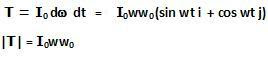## Saturday, March 7, 2009

### Irodov Problem 1.280The entire system including the motor M, counter balance N, sphere A, the platform and so on) is free to move about the axis OO'. Thus, if there
are no external torques to this system
abou the axis OO' then the angular momentum about the axis OO' will be conserved. Since, the motor and all its actions are internal to this system, the forces/torques exerted by the motor do not contrtibute towards any change in angular momentum of the system about the axis OO'.

Initially the sphere is spinning about axis BB' (aligned with OO') with angular velocity wo. The moment of inertia of the sphere about BB' is Io. The rest of the system is not spinning and thus has no angular momentum about OO'.Thus, the angular momentum of the entire system about OO' is simply the angular momentum of the sphere given by Iowo. In vector notation the angular momentum vector will be along the z-axis thus we can write the initial angular mometum of the entire system as Iowo k.

If a rigid body has an angular velocity vectorthen , its kinetic energy is given by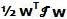where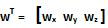(the matrix transpose), and the moment of inertia tensor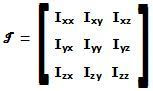. The various components of the moment of inertia tensor can be calculated by knowing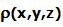, the density of the rigid body at location (x,y,z) as,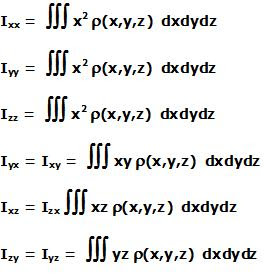For the sphere, if we consider as the center of the sphere as the origin then,.
So, is the sphere's angular velocity isthen the kinetic energy of the sphere will be given by.

The rest of the system including the platform, the motor, the counter balance etc can only rotate about the axis OO' and have a moment of inertia I. Thus, the kinetic energy of this part of the system will be simply.

a)After the motor turns the sphere system by 90 degrees as shown in the figure, the sphere now rotates about the axis BB' (which has turned by 90 degrees) with and angular velocity wo. Thus, its angular momentum about the axis BB' which is now perpendicular to the axis OO' (perpendicular to the screen) is given by -Iowo j oriented along the axis BB'.

The initial angular momemtum of the system was however along the z-axis. This means that the only way angular momentum of the entire system about OO' (which was Iowo k) can be conserved is if the entire system starts to spin about the axis OO' . Let us assume that the entire system aquires a an angular velocity w about OO' by the time the motor has turned 90 degrees. The moment of inertia of the entire system is I+Io. Thus, we have,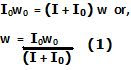The total kinetic energy of the system is the sum of i) kinetic energy of the sphere and ii) the kinetic energy of the rest of the system. Let us first find the kinetic energy of the sphere. The sphere is subject to two kinds of spinning motions, a) about BB' at a rate w0 and b) at a rate OO' with angular velocity w given by (1) along with the entire system. The net angular velocity vector of the sphere can thus be written as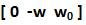. Hence, its kinetic energy, as discussed before, is given by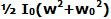. The rest of the system is roating about the axis OO' at a rate w given by (1) and so its kinetic energy is given by. The net kinetic energy of the entire system is thus given by.

The work done by the motor in turning the sphere by 90 degrees is thus entirely responsible for the increase in rotational kinetic energy of the system. The initial kinetic energy of the system was simply the kinetic energy of the rotating sphere given by.
The work done by the motor is thus given by,When the sphere has been turned 180 degrees, its now essentially spinning in the opposite direction to what it was spinning initially. Its angular momentum now is thus -wo (the angular velocity vector directed along the negative z-axis to concide with the inverted axis BB') . Let us suppose that the rest of the system is rotating with an angular velocity w about the axis OO'. For conservation of angular momentum we have,The total kinetic energy of the system is the sum of the kinetic energy of the sphere given byand that of the rest of the system given by, w given in (2). The change in kinetic energy is the work done by the motor and is given by,b)When the sphere is in the 90 degrees position, the sphere is subject to two kinds of spinning motion i) about BB' at a rate w0 and ii) at a rate OO' with angular velocity w given by (1) along with the entire system. This combined motion can be essentially explained as the angular velocity vector wo rotating in the x-y plane at a constant angular velocity w.

Thus, we can write the angular velocity of the sphere also as,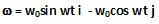This means that the angular velocity vector of the sphere is constantly changing. Any change in angular velocity vector has to be provided by a torque (moment) equal to the rate of change of angular momentum of the body. This moment is given by,